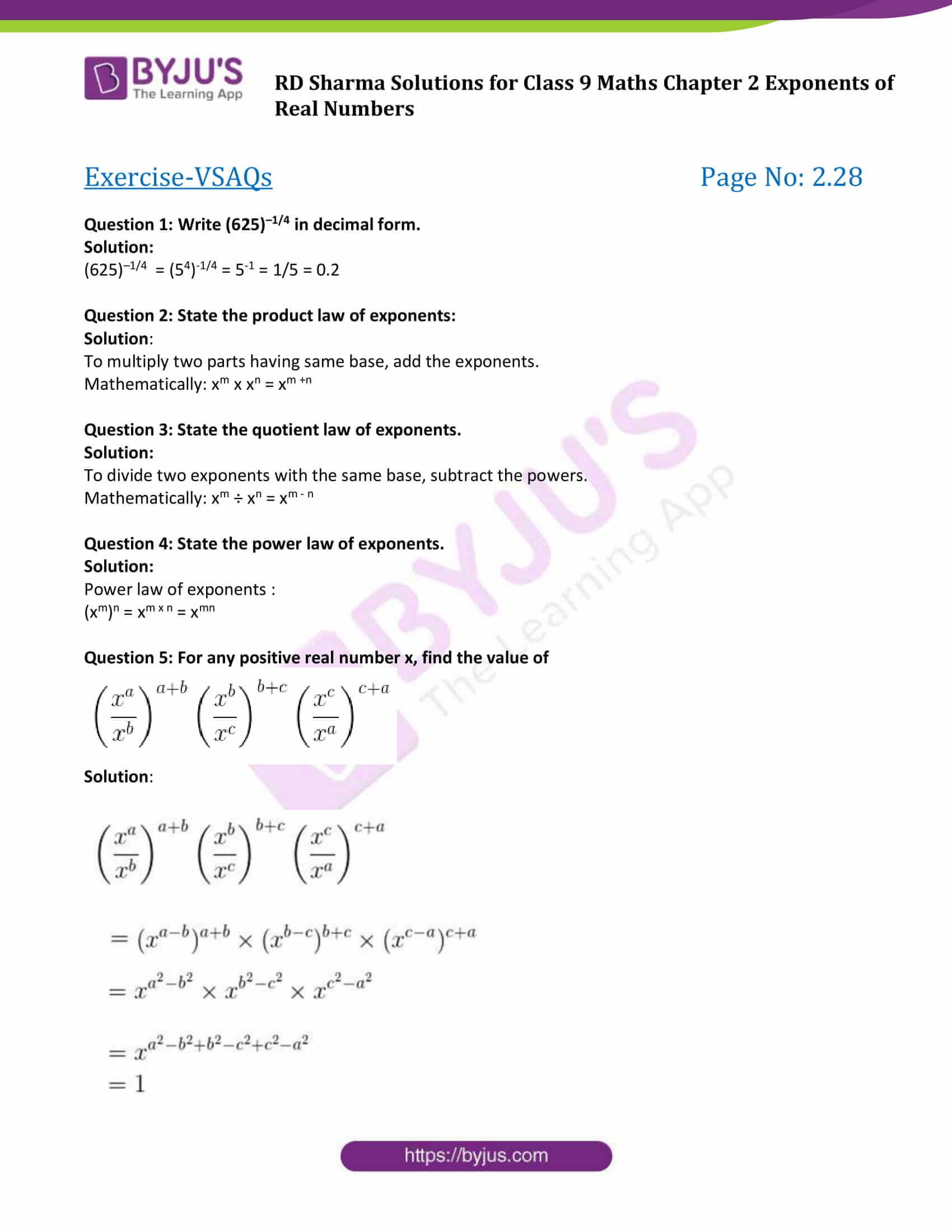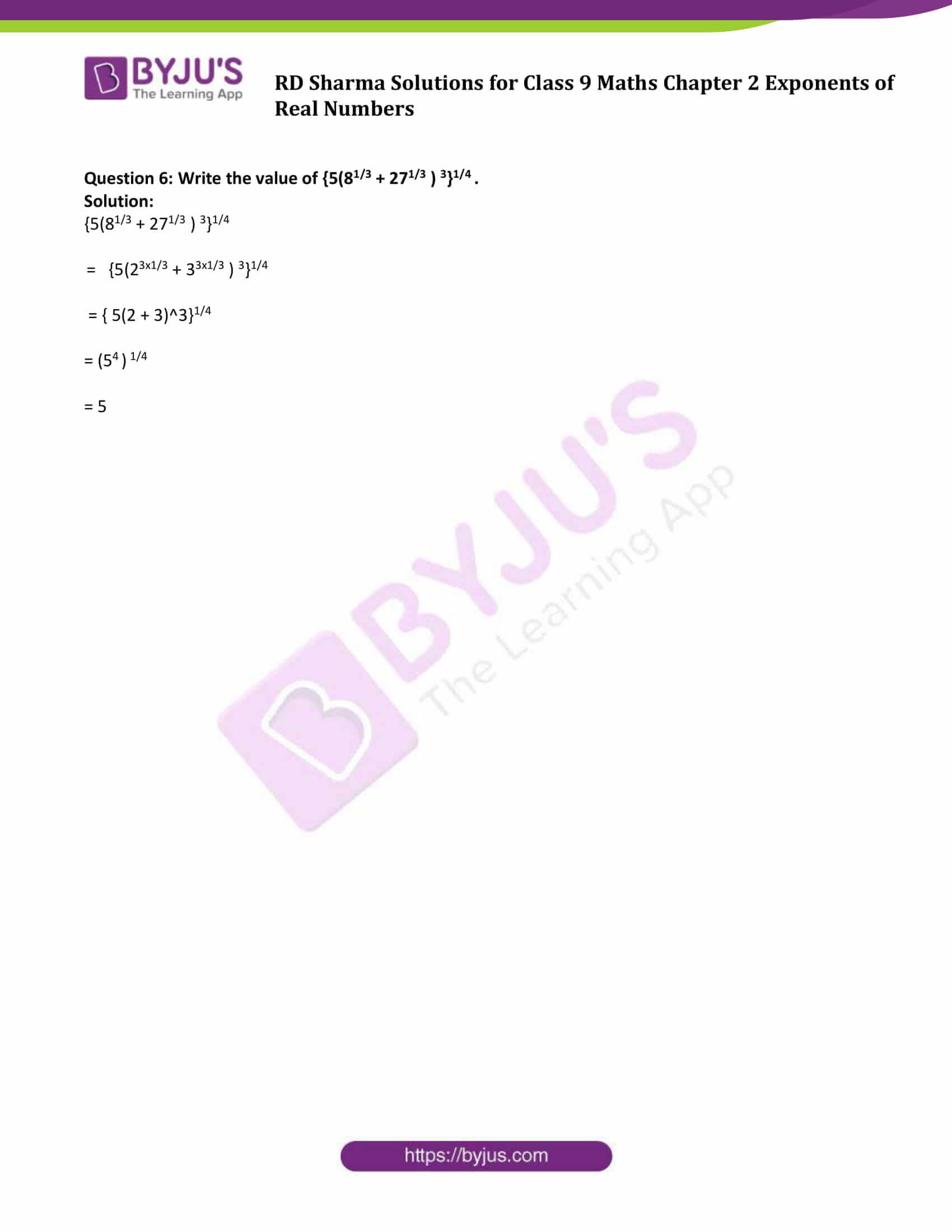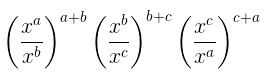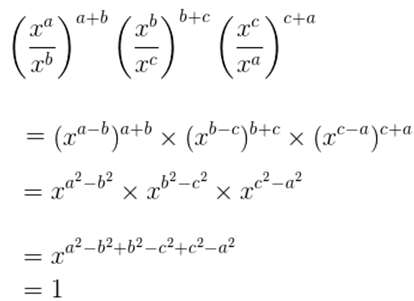# RD Sharma Solutions for Class 9 Maths Chapter 2 Exponents of Real Numbers Exercise VSAQs

RD Sharma Class 9 Mathematics Chapter 2 Exercise VSAQs Exponents of Real Numbers is provided here. This exercise is about laws of integral exponents and nth root of a positive real number and many more. RD Sharma Solutions Class 9 chapter 2 are provided by BYJU’S for students of 9th standard to help them score good marks. Click on the link to download free PDF of chapter 2 exercise VSAQs.

## Download PDF of RD Sharma Solutions for Class 9 Maths Chapter 2 Exponents of Real Numbers Exercise VSAQs### Access Answers to Maths RD Sharma Solutions for Class 9 Chapter 2 Exponents of Real Numbers Exercise VSAQs

Question 1: Write (625)–1/4 in decimal form.

Solution:

(625)–1/4 = (54)-1/4 = 5-1 = 1/5 = 0.2

Question 2: State the product law of exponents:

Solution:

To multiply two parts having same base, add the exponents.

Mathematically: xm x xn = xm +n

Question 3: State the quotient law of exponents.

Solution:

To divide two exponents with the same base, subtract the powers.

Mathematically: xm ÷ xn = xm – n

Question 4: State the power law of exponents.

Solution:

Power law of exponents :

(xm)n = xm x n = xmn

Question 5: For any positive real number x, find the value ofSolution:Question 6: Write the value of {5(81/3 + 271/3 ) 3}1/4 .

Solution:

{5(81/3 + 271/3 ) 3}1/4

= {5(23×1/3 + 33×1/3 ) 3}1/4

= { 5(2 + 3)^3}1/4

= (54 ) 1/4

= 5

## RD Sharma Solutions for Class 9 Maths Chapter 2 Exponents of Real Numbers Exercise VSAQs

RD Sharma Solutions Class 9 Maths Chapter 2 Exponents of Real Numbers Exercise VSAQs is based on the following topics:

• Rational Exponents of Real Number
• nth root of a positive real number
• Laws of rational exponents